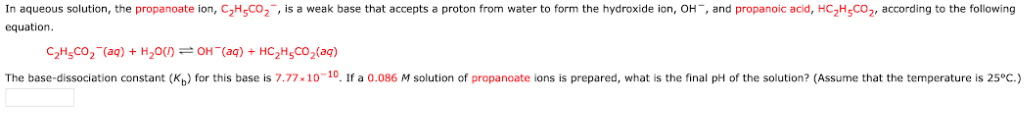# In aqueous solution, the propanoate ion, C2H5CO2-, is a weak base that accepts a proton from water to form the hydroxide ion, OH-, and propanoic acid, HC2H5CO2, according to the following equation. C2H5CO2-(aq) + H2O(l) = OH-(aq) + HC2H5CO2(aq) The base-dissociation constant (Kb) for this base is 7.77 x 10^-10. If a 0.086 M solution of a propanoate ions is prepared, what is the final pH of the solution? (Assume that the temperature is 25°C.)